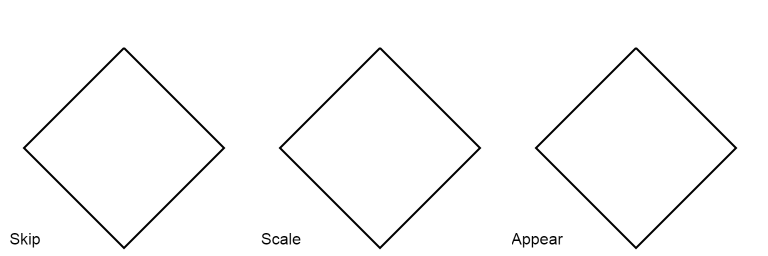### ImageProcessing.Compute.Path.Interpolate

This node interpolates between two SVG paths whereby the path data is specified via the path A and path B text input slots. The SVG path data format can be found here. The interpolation is controlled via the weight slot with a value within the range from 0.0 to 1.0. A weight of 0.0 returns path A and a weight of 1.0 path B. Intermediate values create the corresponding linear interpolation of both paths.

If both paths have the same number of subpaths, each subpath pair from A and B is interpolated individually. Thereby it is possible that the subpaths have different types, e.g. a line can be interpolated to a Bézier curve, a quadratic Bézier curve to a cubic Bézier curve, etc. This is achieved by a degree elevation of the subpath that has a lower degree.

If both paths have not the same number of subpaths, there are different options, which can be selected via the enlarge mode slot. If the enlarge mode is "skip" the additional subpaths are not included in the output. If the enlarge mode is "scale", the additional subpaths are interpolated with the last point where the shorter path ends. If the enlarge mode is "appear", the additional subpaths are appearing in linear relation to the given interpolation weight. This behavior is illustrated in the figures below.The example PathInterpolation demonstrates the usage of this node.